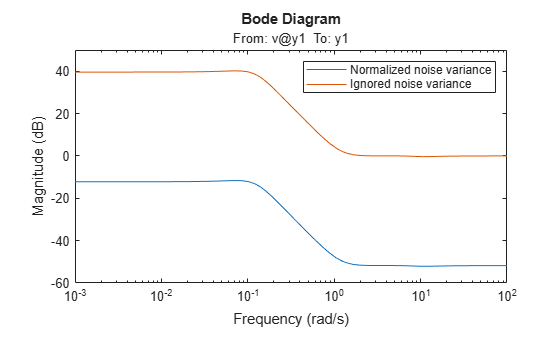Main Content

# noise2meas

Noise component of model

## Syntax

```noise_model = noise2meas(sys) noise_model = noise2meas(sys,noise) ```

## Description

```noise_model = noise2meas(sys)``` returns the noise component, `noise_model`, of a linear identified model, `sys`. Use `noise2meas` to convert a time-series model (no inputs) to an input/output model. The converted model can be used for linear analysis, including viewing pole/zero maps, and plotting the step response.

```noise_model = noise2meas(sys,noise)``` specifies the noise variance normalization method.

## Input Arguments

 `sys` Identified linear model. `noise` Noise variance normalization method, specified as one of the following values: `'innovations'` — Noise sources are not normalized and remain as the innovations process.`'normalize'` — Noise sources are normalized to be independent and of unit variance. Default: `'innovations'`

## Output Arguments

 `noise_model` Noise component of `sys`. `sys` represents the system `$y\left(t\right)=Gu\left(t\right)+He\left(t\right)$` G is the transfer function between the measured input, u(t), and the output, y(t). H is the noise model and describes the effect of the disturbance, e(t), on the model’s response. An equivalent state-space representation of `sys` is `$\begin{array}{l}\stackrel{˙}{x}\left(t\right)=Ax\left(t\right)+Bu\left(t\right)+Ke\left(t\right)\\ y\left(t\right)=Cx\left(t\right)+Du\left(t\right)+e\left(t\right)\\ e\left(t\right)=Lv\left(t\right)\end{array}$` v(t) is white noise with independent channels and unit variances. The white-noise signal e(t) represents the model’s innovations and has variance LLT. The noise-variance data is stored using the `NoiseVariance` property of `sys`. If `noise` is `'innovations'`, then `noise2meas` returns H and `noise_model` represents the system`$y\left(t\right)=He\left(t\right)$`An equivalent state-space representation of `noise_model` is`$\begin{array}{l}\stackrel{˙}{x}\left(t\right)=Ax\left(t\right)+Ke\left(t\right)\\ y\left(t\right)=Cx\left(t\right)+e\left(t\right)\end{array}$``noise2meas` returns the noise channels of `sys` as the input channels of `noise_model`. The input channels are named using the format `'e@yk'`, where `yk` corresponds to the `OutputName` property of an output. The measured input channels of `sys` are discarded and the noise variance is set to zero.If `noise` is `'normalize'`, then `noise2meas` first normalizes`$e\left(t\right)=Lv\left(t\right)$``noise_model` represents the system`$y\left(t\right)=HLv\left(t\right)$`or, equivalently, in state-space representation`$\begin{array}{l}\stackrel{˙}{x}\left(t\right)=Ax\left(t\right)+KLv\left(t\right)\\ y\left(t\right)=Cx\left(t\right)+Lv\left(t\right)\end{array}$`The input channels are named using the format `'v@yk'`, where `yk` corresponds to the `OutputName` property of an output. The model type of `noise_model` depends on the model type of `sys`. `noise_model` is an `idtf` model if `sys` is an `idproc` model.`noise_model` is an `idss` model if `sys` is an `idgrey` model.`noise_model` is the same type of model as `sys` for all other model types. To obtain the model coefficients of `noise_model` in state-space form, use `ssdata`. Similarly, to obtain the model coefficients in transfer-function form, use `tfdata`.

## Examples

collapse all

Convert a time-series model to an input/output model that may be used by linear analysis tools.

Identify a time-series model.

```load iddata9 z9 sys = ar(z9,4,'ls');```

`sys` is an `idpoly` model with no inputs.

Convert `sys` to a measured model.

`noise_model = noise2meas(sys);`

`noise_model` is an `idpoly` model with one input.

You can use `noise_model` for linear analysis functions such as `step`, `iopzmap`, etc.

Convert an identified linear model to an input/output model, and normalize its noise variance.

Identify a linear model using data.

```load twotankdata; z = iddata(y,u,0.2); sys = ssest(z,4);```

`sys` is an `idss` model, with a noise variance of 6.6211e-06. The value of $L$ is `sqrt(sys.NoiseVariance)`, which is 0.0026.

View the disturbance matrix.

`sys.K`
```ans = 4×1 0.2719 1.6570 -0.6318 -0.2877 ```

Obtain a model that absorbs the noise variance of `sys`.

`noise_model_normalize = noise2meas(sys,'normalize');`

`noise_model_normalize` is an `idpoly` model.

View the $B$ matrix for `noise_model_normalize`

`noise_model_normalize.B`
```ans = 4×1 0.0007 0.0043 -0.0016 -0.0007 ```

As expected, `noise_model_normalize.B` is equal to `L*sys.K`.

Compare the bode response with a model that ignores the noise variance of `sys`.

```noise_model_innovation = noise2meas(sys,'innovations'); bodemag(noise_model_normalize,noise_model_innovation); legend('Normalized noise variance','Ignored noise variance');```The difference between the bode magnitudes of the `noise_model_innovation` and `noise_model_normalized` is approximately 51 dB. As expected, the magnitude difference is approximately equal to `20*log10(L)`.

## See Also

Introduced in R2012a

## SupportGet trial now